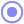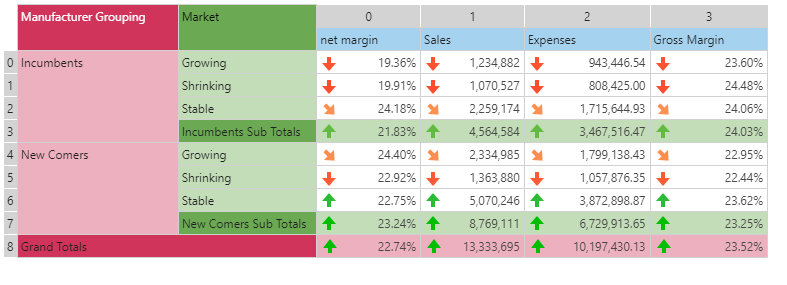#IfNull (PQL)

Evaluates if an expression resolves to NULL, returning the an alternative value if true, otherwise returning the original value.

• Returned Output: Numeric
• Library: PQL \ Common \ Logical
• Version: 2018.00.000
• Compatibility:
• Can be combined with and other PQL function throughout the application.
• It CANNOT be used with MDX or VBA functions. But it can be used on MDX-based content in other parts of the application.

#### Syntax

IfNull(, <Numeric> )

* Click on the function's arguments above for more details on the input values.

• The expression should evaluate to a numeric result
• The alternative value can be any alphanumeric value or embedded function that resolve to a value.
##### Different Function types
• This function is like the Excel "IsEmpty" function.
• Also see the If function.

#### Examples

This example uses the dynamic grid below.

IfNull( data(0,0), 12.8 )

The function checks if the value of cell 0,0 is null. If it is, it returns 12.8. In this scenario it isn't, so it returns 19.36%